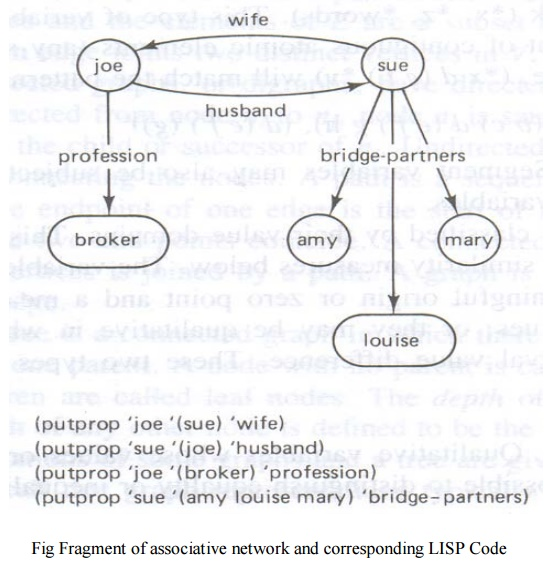Home | | Artificial Intelligence | Structures used in Matching

# Structures used in Matching

The types of list structures represent clauses in propositional or predicate logic such as (or ~(MARRIED ?x ?y) ~(DAUGHTER ?z ?y) (MOTHER ?y ?z)) or rules such as (and ((cloudy-sky) (low-bar-pressure) (high-humidity)) (conclude (rain likely)) or fragments of associative networks in below Fig

Structures used in Matching

The types of list structures represent clauses in propositional or predicate logic such as (or ~(MARRIED ?x ?y) ~(DAUGHTER ?z ?y) (MOTHER ?y ?z)) or rules such as (and ((cloudy-sky) (low-bar-pressure) (high-humidity)) (conclude (rain likely)) or fragments of associative networks in below Fig

The other common structures include strings of characters a1 a2 . . . ak , where the ai belong to given alphabet A, vector X = (x1 x2 . . . xn), where the xi represents attribute values, matrices M (rows of vectors), general graphs, trees and setsVariables

The structures are constructed from basic atomic elements, numbers and characters

Character string elements may represent either constants or variables

If variables, they may be classified by either the type of match permitted or their value domains

An open variable can be replaced by a single item

Segment variable can be replaced by zero or more items

Open variable are replaced with a preceding question mark (?x. ?y, ?class)

They may match or assume the value of any single string element or word, but they are subject to consistency constraints

For example, to be consistent, the variable ?x can be bound only to the same top level element in any single structure

Thus (a ?x d ?x e) may match (a b d b e), but not (a b d a e)

Segment variable types will be preceded with an asterisk (*x, *z, *words)

This type of variable can match an arbitrary number or segment of contiguous atomic elements

For example, (* x d (e g) *y) will match the patterns

(a (b c) d (e f) g h), (d (e f ) (g))

Subject variable may also be subject to consistency constraints similar to open variables

Nominal variables

Qualitative variables whose values or states have no order nor rank

It is possible to distinguish between equality or inequality between two objects

Each state can be given a numerical code

For example, â€śmarital statusâ€ť has states of married, single, divorced or widowed. These states could be assigned numerical codes, such as married = 1, single = 2, divorced = 3 and widowed = 4

Ordinal variables

Qualitative variables whose states can be arranged in a rank order

It may be assigned numerical values

Foe example, the states very tall, tall, medium, short and very short can be arranged in order from tallest to shortest and can be assigned an arbitrary scale of 5 to 1

Binary variable

Qualitative discrete variables which may assume only one of two values, such as 0 or 1, good or bad, yes or no, high or low

Interval variables or Metric variables

Qualitative variables which take on numeric values and for which equal differences between values have the same significance

For example, real numbers corresponding to temperature or integers corresponding to an amount of money are considered as interval variables

Graphs and Trees

A graph is a collection of points called vertices, some of which are connected by line segments called edges

Graphs are used to model a wide variety of real-life applications, including transportation and communication networks, project scheduling, and games

A graph G = (V, E) is an ordered pair of sets V and E. the elements V are nodes or vertices and the elements of E are a subset of V X V called edges

An edge joints two distinct vertices in V

Directed graphs or digraphs, have directed edges or arcs with arrows

If an arc is directed from node ni to nj , node ni is said to be a parent or successor of nj and nj is the child or successor of ni

Undirected graphs have simple edges without arrows connecting the nodes

A path is a sequence of edges connecting two modes where the endpoint of one edge is the start of its successor

A cycle is a path in which the two end points coincide

A Connected graph is a graph for which every pair of vertices is joined by a path

A graph is complete if every element of V X V is an edge

A tree is a connected graph in which there are no cycles and each node has at most one parent below

A node with no parent is called root node

A node with no children is called leaf node

The depth of the root node is defined as zero

The depth of any other node is defined to be the depth of its parent plus 1Sets and Bags

A set is represented as an unordered list of unique elements such as the set (a d f c) or (red blue green yellow)

A bag is a set which may contain more than one copy of the same member a, b, d and e

Sets and bags are structures used in matching operations

Study Material, Lecturing Notes, Assignment, Reference, Wiki description explanation, brief detail
Artificial Intelligence : Structures used in Matching |